# 3530. 和你在一起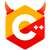$$let\,\,a\,\,be\:\:321\;\;and\;\;b\;\;be\;\;42$$
$$we\;\;have$$
$$a+b=32142<b+a=42321$$

#include <algorithm>
#include <iostream>
#include <string>

using namespace std;

int main() {
int n;
cin.tie(0);
ios::sync_with_stdio(0);
cin >> n;
string *nums = new string[n], *ed = nums + n;
for (string *i = nums; i != ed; ++i)
cin >> *i;
sort(nums, ed, [](string &a, string &b) { return b + a < a + b; });
for (string *i = nums; i != ed; ++i)
cout << *i;
cout << '\n';
}EOJ 的 Markdown 也太丑了int main() {
unsigned n; cin >> n;
vector<string> nums(n);
for (auto &i : nums)
cin >> i;
sort(nums.begin(), nums.end(), [](string const& a, string const& b) { return b + a < a + b; });
for (auto const& i : nums)
cout << i;
}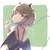3
78 71 7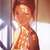#include<iostream>
#include<algorithm>
using namespace std;
int fun(string a, string b){
if(a.length() == b.length()){
//do nothing
}
else if(a.length() > b.length()){
int dlen = a.length() - b.length();
for(int i = 0; i < dlen; i++){
b += a[i];
}
}
else{
int dlen = b.length() - a.length();
for(int i = 0; i < dlen; i++){
a += b[i];
}
}
return a > b;
}
int main(){
int n;
string arr;
cin >> n;
for(int i = 0; i < n; i++){
cin >> arr[i];
}
sort(arr, arr + n, fun);
for(int i = 0; i < n; i++){
cout << arr[i];
}
return 0;
}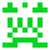bool EOJ_3530_cmp(string a, string b)
{
return a + b > b + a; //a在前比较大时，把a放在b前面
}
int main()
{
int N;
cin >> N;
vector vi;

string temp;
for (int i = 0; i < N; i++)
{
cin >> temp;
vi.push_back(temp);
}
sort(vi.begin(), vi.end(), EOJ_3530_cmp);

for (int i = 0; i < N; i++)
cout << vi[i];
cout << endl;
return 0;


}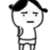n = int(input())
x=list(input().split())
x.sort(reverse=True)
for m in x:
print(m,end='')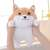#include<stdio.h>
#include<math.h>
#include<stdlib.h>
#include<string.h>

struct num{
int mynum;
};
typedef struct num NUM;

int mycoup(const void * p1,const void * p2);
int min(int a,int b);

int main(void)
{
int cases,i;
NUM *inputlst;

scanf("%d",&cases);
inputlst=(NUM*)malloc(cases*sizeof(NUM));

for(i=0;i<cases;i++)
scanf("%d",&inputlst[i].mynum);

qsort(inputlst,cases,sizeof(NUM),mycoup);
for(i=0;i<cases;i++)
{
printf("%d",inputlst[i].mynum);
if(i==cases-1)
printf("\n");
}

free(inputlst);
return 0;
}

int mycoup(const void * p1,const void * p2)
{
const NUM * a1=(const NUM *)p1;
const NUM * a2=(const NUM *)p2;
char temp1,temp2;
int i,len1,len2;

sprintf(temp1,"%d",a1->mynum);
sprintf(temp2,"%d",a2->mynum);

len1=strlen(temp1);
len2=strlen(temp2);

for(i=0;i<min(len1,len2);i++)
{
if(temp1[i]>temp2[i])
return -1;
else if(temp1[i]<temp2[i])
return 1;
}
return 0;
}

int min(int a,int b)
{
if(a<b)
return a;
else
return b;
}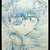### include

using namespace std;
typedef struct{
char num;
}ST;
ST s;

bool cmp(ST a,ST b){
return strcmp(a.num,b.num)>0;
}

int main(){
int n,i;
cin>>n;
for(i=0; i>s[i].num;
}
sort(s,s+n,cmp);
for(i=0; i<n; i++){
cout<<s[i].num;
}
return 0;
}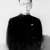AC输出：912978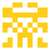input()
print(‘’.join(sorted(input().split(‘ ‘), reverse=True)))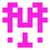### include

using namespace std;
int main()
{
int n;
int a = {};
int b = {};
int c = {};
cin >> n;
for (int i = 0; i < n; i++)
{
cin >> a[i];
}
for (int i = 0; i < n; i++)
{
if (a[i] >1000){ b[i] = a[i] / 1000; }
else if (a[i] >100 && a[i] < 1000)
{
b[i] = a[i] / 100;
}

    else if (a[i] >= 10 && a[i] <= 100)
{
b[i] = a[i] / 10;
}
else
b[i] = a[i] % 10;
}
int temp;
for (int i = 0; i < n; i++)
{
for (int j = i; j < n; j++)
{
if (b[j]>b[i])
{
temp = b[i]; b[i] = b[j]; b[j] = temp;
temp = a[i]; a[i] = a[j]; a[j] = temp;
}
if (b[j]==b[i])
{
if (a[j]<10)
{
temp = b[i]; b[i] = b[j]; b[j] = temp;
temp = a[i]; a[i] = a[j]; a[j] = temp;
}
if (a[j]>100 && a[j] < 1000)
{
if (a[i]>1000){
if (a[j] > (a[i] / 10))
{
temp = b[i]; b[i] = b[j]; b[j] = temp;
temp = a[i]; a[i] = a[j]; a[j] = temp;
}

}
}
if (a[i]>100 && a[i] < 1000)
{
if (a[j]>1000){
if (a[j] / 10 > (a[i]))
{
temp = b[i]; b[i] = b[j]; b[j] = temp;
temp = a[i]; a[i] = a[j]; a[j] = temp;
}

}
}
if ((a[j] > 1000 && a[i] > 1000) ||( (a[j] < 1000 && a[j]>100) && (a[i] < 1000 && a[i]>100)))
{
if (a[j]>a[i])
{
temp = b[i]; b[i] = b[j]; b[j] = temp;
temp = a[i]; a[i] = a[j]; a[j] = temp;
}
}
}

}
c[i] = a[i];
}
for (int i = 0; i < n; i++){ cout << c[i]; }


}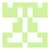### include

using namespace std;
typedef struct Data{
int num;
int first;
int second;
int third;
int forth;
}data;
bool cmp(Data a, Data b){
if(a.first == b.first){
if(a.second == b.second){
if(a.third == b.third){
return a.forth > b.forth;
}
return a.third > b.third;
}
return a.second > b.second;
}
return a.first > b.first;
}
int main()
{
int n;
cin >> n;
int i;
Data data[n];
for(i = 0; i < n; i++){
int number;
cin >> number;
data[i].num = number;
int fir, sed, thid, fort;
fir = number / 1000;
number = number % 1000;
sed = number / 100;
number = number % 100;
thid = number / 10;
fort = number % 10;
while(fir == 0){
fir = sed;
sed = thid;
thid = fort;
fort = fort;
}
data[i].first = fir;
data[i].second = sed;
data[i].third = thid;
data[i].forth = fort;
}
sort(data, data + n, cmp);
for(i = 0; i < n; i++){
cout << data[i].num;
}
cout << endl;
return 0;
}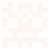### include

using namespace std;

bool cmp(string a,string b){
return a+b>b+a;
}

int main(){
int n;
cin>>n;
vector vec;
string input;
for(int i=0;i>input;
vec.push_back(input);
}
sort(vec.begin(),vec.end(),cmp);
for(vector::iterator it=vec.begin();it!=vec.end();it++)
cout<< *it;
return 0;
}